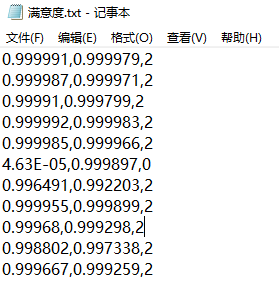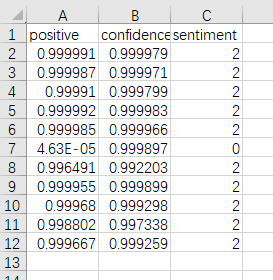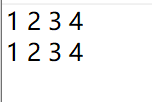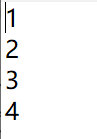• file2 = open(filename + '.txt', 'w') for i in range(len(list1)): for j in range(len(list1[i])): file2.write(str(list1[i][j])) # write函数不能写int类型的参数，所以使用str()转化 file2.write('...
def Save_list(list1,filename):
file2 = open(filename + '.txt', 'w')
for i in range(len(list1)):
for j in range(len(list1[i])):
file2.write(str(list1[i][j]))              # write函数不能写int类型的参数，所以使用str()转化
file2.write('\t')                          # 相当于Tab一下，换一个单元格
file2.write('\n')                              # 写完一行立马换行
file2.close()
————————————————
版权声明：本文为CSDN博主「柒一水」的原创文章，遵循CC 4.0 BY-SA版权协议，转载请附上原文出处链接及本声明。
原文链接：https://blog.csdn.net/qq_28156907/article/details/103120247

展开全文• python 处理txt 为 csv文件 例如将文本文档中，如下数据存入csv中。 其中第一例代表positive，第二列confidence，第三列sentiment 第一步：先打开满意度.txt文件夹，将数据处理为列表的格式 with open("满意度....
python 处理txt 存为 csv文件
例如将文本文档中，如下数据存入csv中。
其中第一例代表positive，第二列confidence，第三列sentiment第一步：先打开满意度.txt文件夹，将数据处理为列表的格式
with open("满意度.txt", "r", encoding="utf8") as f:
# 对文本文件按行读取
read = f.readlines()
# 新建空列表
anaylize = []
for row in read:
# 先去掉每一列中的换行符，然后以逗号分隔为数据
data = row.strip("\n").split(",")
# 将列表转化为字典的格式
anaylize_dict = {'positive': data,
'confidence': data,
'sentiment': data}
# 将字典append空列表上
anaylize.append(anaylize_dict)

第二部：将列表写入文件
import csv
# newline=""的存在是解决写一行空一行的问题
with open("满意度.csv", "w", encoding="utf8", newline="") as ff:
# csv的表头
field_names = ['positive', 'confidence', 'sentiment']
# 以字典的方式写入文件
writer = csv.DictWriter(ff, field_names)
writer.writeheader()
# 按行写入
writer.writerows(anaylize)

结果如下图所示：展开全文列表 csv
• python读取txt文件为邻接矩阵 import numpy as np def getdata(filename): linedata = open("socfb-Amherst41.txt", 'r') #读取txt文件 cnt = 0 res = [] #读取后的数据，二元列表 n = 2235 matrix = np....
python读取txt文件存为邻接矩阵
import numpy as np
def getdata(filename):

linedata = open("socfb-Amherst41.txt", 'r')    #读取txt文件
cnt = 0
res = []        #存读取后的数据，二元列表
n = 2235
matrix = np.zeros((n, n))
for line in linedata:
linelist = [int(s) for s in line.split()] #每一行根据分割后的结果存入列表
res.append([])
for x in linelist:
res[cnt].append(x)
matrix[max(res[cnt]) - 1][min(res[cnt]) - 1] = 1
matrix[min(res[cnt]) - 1][max(res[cnt]) - 1] = 1
cnt += 1
print(matrix)
return res

filename = 'socfb-Amherst41.txt'
data = getdata(filename)

输出结果就是一个邻接矩阵展开全文列表
• 1.读取txt文件为numpy一维二维数组（loadtxt） import numpy as np #读取并存为数组 a=np.loadtxt('myfile.txt') 2.数组保存到txt文件中（savetxt） import numpy as np a=np.array([[1,2,3,4],[1,2,3,4]]) np...
1.读取txt文件存为numpy一维二维数组（loadtxt）
import numpy as np
#读取并存为数组
a=np.loadtxt('myfile.txt')

2.数组保存到txt文件中（savetxt）
     import numpy as np
a=np.array([[1,2,3,4],[1,2,3,4]])
np.savetxt('myfile.txt', a, fmt="%d", delimiter=" ")

myfile.txt：保存的文件路径以及TXT文本名
a： 为要保存的数组名a
fmt="%d"： 为指数组a以十进制形式保存
delimiter=" "分隔符，此处表示以空格的形式隔开
运行结果但注意，如果数组是一维的，使用savetxt保存时不会保存为一行，而是会以列的形式多行保存：
     import numpy as np
a=np.array([1,2,3,4])
np.savetxt('myfile.txt', a, fmt="%d", delimiter=" ")

运行结果如果在意这种形式，解决方法是：一维数组要间接存为txt，如果是纯数组，直接当成字符串读取并存入txt中
import numpy as np
data = open('IM2.txt', 'w')#打开一个文件，可写
a = np.array([1, 2, 3, 4])
data.write(' '.join(str(i) for i in a))#以' '空格为分隔符将数组各元素以str形式加入文件中
data.close()#关闭文件

运行结果展开全文• 下面是python读取txt里的json文件，到excel的一个小例子。 题目： 纯文本文件 city.txt为城市信息, 里面的内容（包括花括号）如下所示：{ "1" : "上海", "2" : "北京", "3" : "成都"}源码： # -*- coding:...
• python 实现将TXT文件内容逐行到EXCEL，简单易懂，适合初学者
• 实际中，很多数据都是txt文件、csv文件等，但是在程序中处理的时候numpy数组或列表是最方便的。本文简单介绍读入txt文件以及将之转化为numpy数组或列表的方法。1 将txt文件读为list并转化为numpy数组import ...
• 实际中，很多数据都是txt文件、csv文件等，但是在程序中处理的时候numpy数组或列表是最方便的。本文简单介绍读入txt文件以及将之转化为numpy数组或列表的方法。1 将txt文件读为list并转化为numpy数组import ...
• 纯文本文件 numbers.txt, 里面的内容（包括方括号）如下所示：[ [1, 82, 65535], [20, 90, 13], [26, 809, 1024]]用json处理文件 # -*- coding:utf-8 -*- import xlwt,json with open('number.txt','r')as ...
•  #python的字典对应json格式，json.dumps将字典转换成json  json_file_test.write(json.dumps(json_dict)+"\n")  if i == 4 or i == 5 or i == 6 or i == 7 or i == 8 or i == 9 or i == 10 or i == 11:  ...json 正则
• python读取txt天气数据并使用...将txt中的数据逐行到列表lines里 lines的每一个元素对应于txt中的一行。然后将每个元素中的不同信息提取出来： python读取txt天气数据并使用matplotlib模块绘图 这样，所有的信...列表 编程语言 数据挖掘
• (1) 将Python中的数据txt文档中 import numpy as np np.savetxt("/.../test.txt", traindata) (2) Matlab将.mat数据txt文档中 save('/.../test.txt','traindata','-ascii'); (3) Python读取...
• 前言前言：想写这个代码的原因是因为实习的时候需要根据表格名创建对应的文件夹，如果只是很少个数文件夹的话，ctrl+shift+n还可以接受吧，可是一次就要创建几百个文件夹，这就有点方方了。...try.txt...
• Python 把字典里的内容txt with open(savepic+'\\params.txt', 'w') as f: for key, value in params.items(): f.write(key) f.write(': ') f.write(str(value)) ...字典
• 前言 前言：想写这个代码的原因...try.txt的所有要生成的文件夹名称列表，是直接从excel表格获取复制粘贴的，编码格式utf-8；folder文件夹存放的是新生成的目标文件夹，我觉得这样方便点，所以就这么来了。因为只是
• 格式倒是很整齐，就是吧，想一个个数字读出来成列表不知道应该咋整。 姐那么热心的人，当然是亲自试验帮他解决问题了。于是在经过n次调试n次报错被各种数据类型搞晕之后，终于还是挽回了自己的面子给狗比做了出来...数据处理
• fp = open(fname+'.txt',"a",encoding='utf-8') fp.write(section_name+'\n') fp.write(section_text+'\n') fp.close() nexturl_num = content.find("div", class_='bottem1').find_all('a') nexturl_num = nexturl...
• 文章目录1 Segy 格式文件介绍2 Python 编程实现思路3 Python 代码1.引入库2.读入数据总结 1 Segy 格式文件介绍 地震数据处理常用的文件格式为segy格式。 标准segy文件一般包括三部分，第一部分是EBCDIC文件头，长度...
• 今天小编就为大家分享一篇pythontxt等文件中的数据读为numpy数组的方法，具有很好的参考价值，希望对大家有所帮助。一起跟随小编过来看看吧 实际中，很多数据都是txt文件、csv文件等，但是在程序中处理的时候...编程语言
• 实际中，很多数据都是txt文件、csv文件等，但是在程序中处理的时候numpy数组或列表是最方便的。本文简单介绍读入txt文件以及将之转化为numpy数组或列表的方法。1 将txt文件读为list并转化为numpy数组import ......

# python存txtpython 订阅